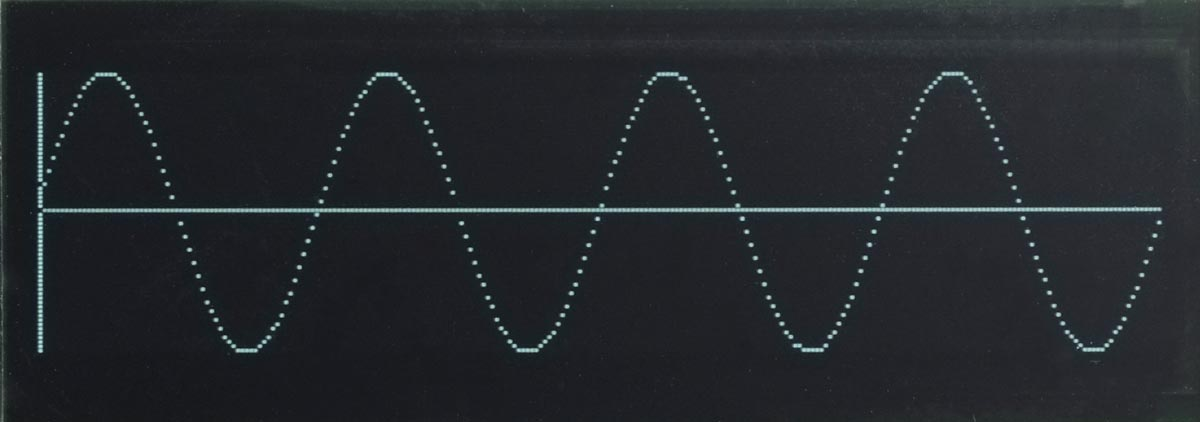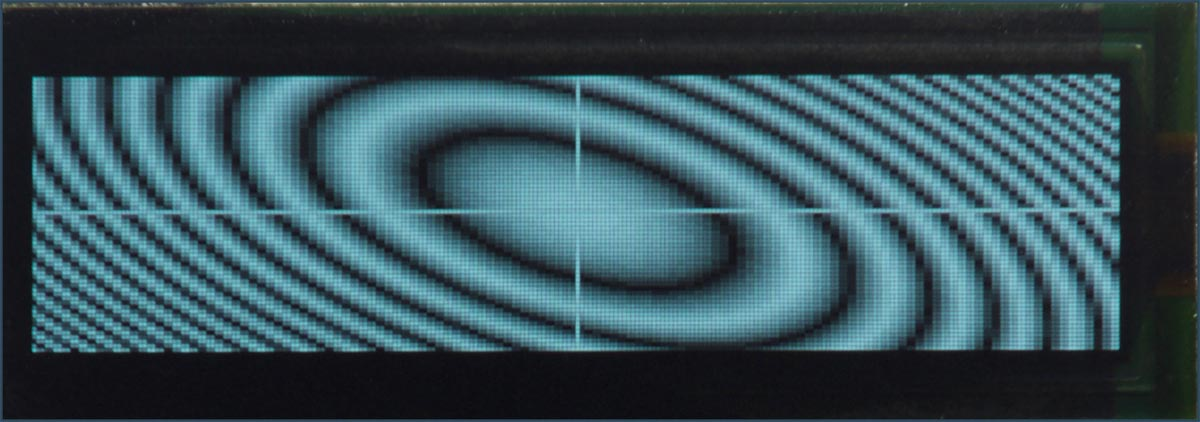## Plotting extensions

This pages lists the plotting extensions included in the version of uLisp for the Lisp Badge.

### Summary

#### Plotting functions

plot, plot3d

After generating a plot both functions wait for the ESC key to be pressed before displaying the uLisp prompt.

### plot function

Syntax: `(plot [x-intercept y-intercept] [function]...)`

Plots up to four functions on the same graph, optionally with axes.

Each function should be a function of one parameter, the x coordinate, and it will be called with each value of x from 0 to 255. The function should return the y value, from 0 to 63.

If x-intercept and y-intercept are specified, plot3d draws axes through those intercepts.

For example, defining:

```(defun sine ()
(let ((x 0) (y 2045))
(lambda (n)
n
(incf x (/ (* y 16) 163))
(decf y (/ (* x 16) 163))
(+ 32 (ash x -6)))))```

the following command:

`(plot 0 32 (sine))`

will plot:#### Plotting multiple functions

The following example plots the voltages on the analogue inputs 0 to 3 once a second on a single plot. First define:

`(defun adc (n) (lambda (x) (delay 250) (/ (analogread n) 8)))`

Then give the command:

`(plot 0 0 (adc 0) (adc 1) (adc 2) (adc 3))`

### plot3d function

Syntax: `(plot3d [x-intercept y-intercept] [function])`

The function should be a function of two parameters, the x and y coordinates, and it will be called with each value of x from 0 to 255 and y from 0 to 63. The function should return the greyscale value to be plotted, from 0 to 15.

If x-intercept and y-intercept are specified, plot3d draws axes through those intercepts.

For example, defining:

```(defun p (x y)
(let ((a (/ (- x 128) 2))
(b (- y 32)))
(min (abs (- (logand (/ (+ (* a a) (* b b) (* a b)) 16) 31) 15)) 15)))```

the following command:

`(plot3d 128 32 p)`

will plot: